# R语言学习笔记—决策树分类

2018/05/02 15:48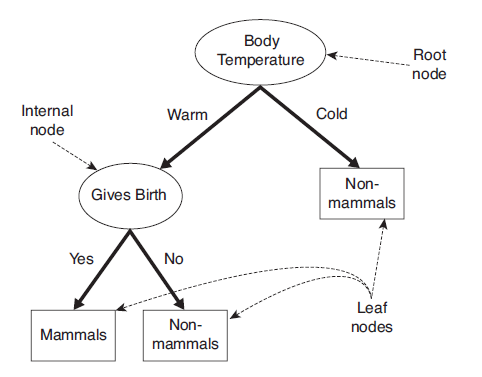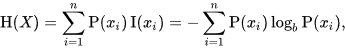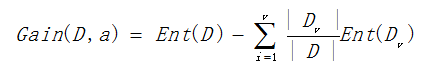1.选择特征属性，样本分割。

2.计算信息增益，选取最大增益作为决策树的子节点。

3.递归执行上两步，直至分类完成。

 Outlook temperature humidity windy play Sunny hot high FALSE no Sunny hot high TRUE no Overcast hot high FALSE yes Rainy mild high FALSE yes Rainy cool normal FALSE yes Rainy cool normal TRUE no Overcast cool normal TRUE yes Sunny mild high FALSE no Sunny cool normal FALSE yes Rainy mild normal FALSE yes Sunny mild normal TRUE yes Overcast mild high TRUE yes Overcast hot normal FALSE yes Rainy mild high TRUE no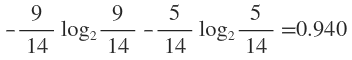outlook temperature humidity windy play yes no yes no yes no yes no yes no sunny 2 3 hot 2 2 high 3 4 FALSE 6 2 9 5 overcast 4 0 mild 4 2 normal 6 1 TRUR 3 3 rainy 3 2 cool 3 1

##决策树模型之ID3算法

#从粘贴板读取表格数据
#查看数据结构
str(weather)
#将windy指标转换成因子型
weather$windy <- as.factor(weather$windy)
#未分割前信息熵
q <- matrix(table(weather$play),nrow = 1,dimnames = list('play',unique(weather$play)))/
sum(matrix(table(weather$play),nrow = 1)) e <- -sum(q*log2(q)) #计算各特征属性的信息熵 myfun <- function(x,y){ t <- table(x,y) m <- matrix(t,nrow = length(levels(x)),2,dimnames = list(levels(x),levels(y))) #权重计算 n <- apply(m,1,sum)/sum(m) #分割后各子集的熵 freq <- -rowSums((m/rowSums(m))*log2(m/rowSums(m))) #分割后的最终熵 entropy <- sum(n*freq,na.rm = T) return(entropy) } #计算信息增益information gain y <- weather[,5] gain <- vector() for (i in 1:(length(weather)-1)) { x <- weather[,i] gain[i] <- e-myfun(x,y) } names(gain) <- colnames(weather[,1:4]) gain 运行结果：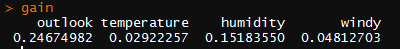根据各特征属性的信息增益比较得到，outlook信息增益最大，即熵变化越大，所以决策树的根节点应选择outlook。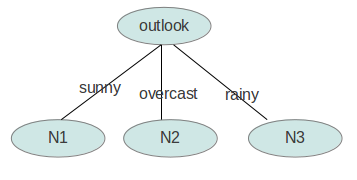接下来选择各子节点N1，N2，N3的特征属性。 用属性outlook划分样本后，三个属性值sunny，overcast，rainy把样本划分为三部分，在每一部分下分别计算gain（temperature）、gain（humidity）、gain（windy） 代码： #选择子节点特征属性 level <- levels(weather$outlook)
son_gain <- data.frame()
for(j in 1:length(level)){
son_q <- matrix(table(weather[weather$outlook==level[j],]$play),nrow = 1,
dimnames = list('play',unique(weather$play)))/ sum(matrix(table(weather[weather$outlook==level[j],]$play),nrow = 1)) son_e[j]<- -sum(son_q*log2(son_q)) } for (j in 1:length(level)) { for (i in 1:length(level)) { sl <- weather[weather$outlook==level[j],]
son_x <- sl[,i+1]
son_y <- sl[,5]
son_gain[j,i] <- son_e[j]-myfun(x=son_x,y=son_y)
}
}
colnames(son_gain) <- colnames(weather[,-c(1,5)])
rownames(son_gain) <- level
son_gain

信息增益结果：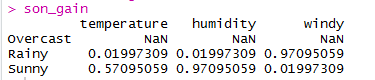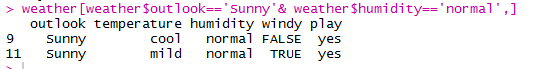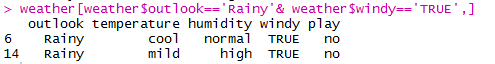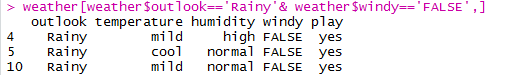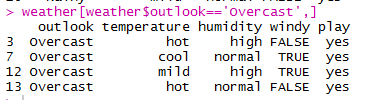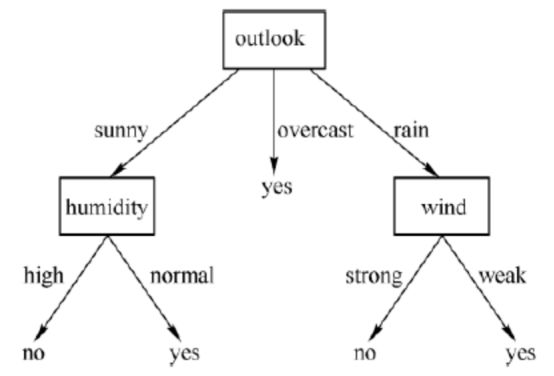0
0 收藏

### 作者的其它热门文章0 评论
0 收藏
0